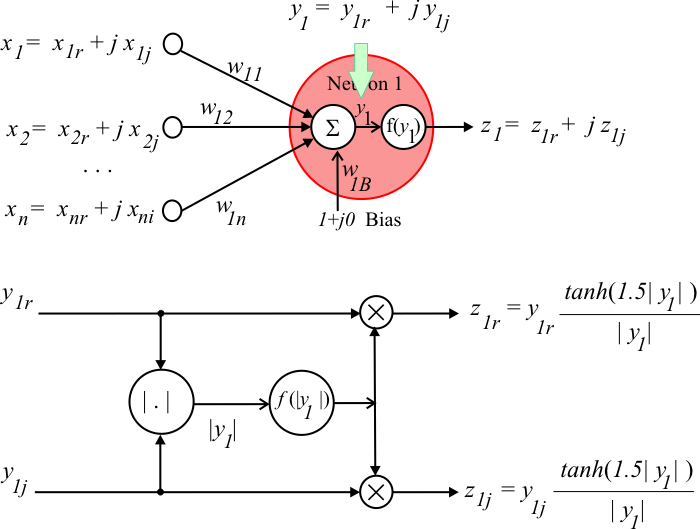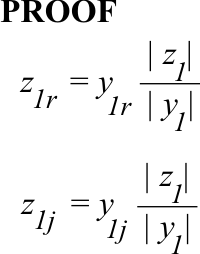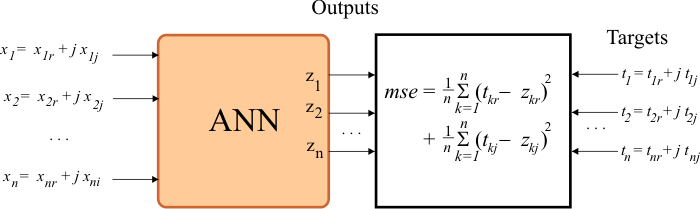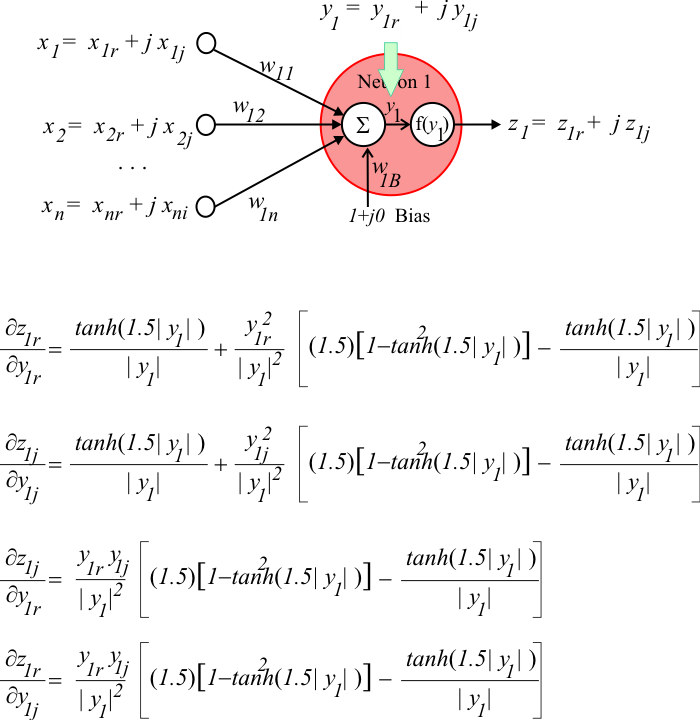# Activation Function

## Activation Function

In a complex domain ANN, each neuron receives several complex input values and produces an output complex value. Thus, the activation of a complex domain neuron is different to the activation of a real domain neuron. The figure below shows how the activation of a complex domain neuron works. The module of y is computed and used produced a scaling factor using the tanh function. Second, the real and imaginary output components are computed as shown.Problem 1 Proof that in a complex domain neuron the output can be expressed as shown.## mse

In a complex domain ANN, the mse must be calculated using the real and imaginary parts of the output and the target as shown below.## Derivatives of the Activation Function

In order to train a complex domain ANN, it is necessary to compute the partial derivatives of the output of the network as shown.